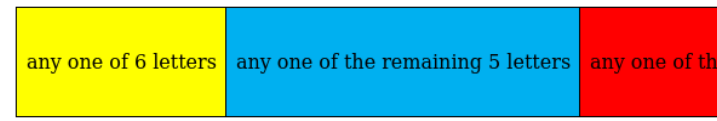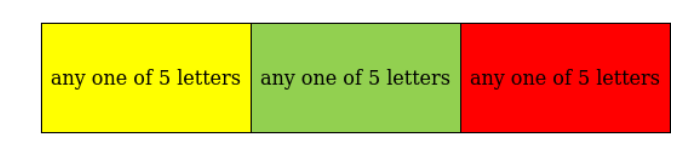Deepak Scored 45->99%ile with Bounce Back Crack Course. You can do it too!

# How many 3-letters words can be formed using a, b, c, d, e if

Question:

How many 3-letters words can be formed using a, b, c, d, e if

(i) Repetition of letters is not allowed?

(ii) Repetition of letters is allowed

Solution:(i) if repetition of letters is not allowed then number of many 3 -letters words that can be formed using $a, b, c, d, e$ are

$5 \times 4 \times 3=60$(ii) if repetition of letters is allowed then number of many 3-letters words that can be formed using $a, b, c, d$, e are

$5 \times 5 \times 5=125$